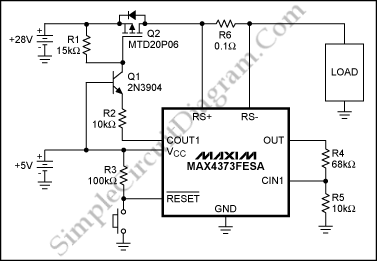# Max4373 Electronic Circuit Breaker

This a high-side current amplifier circuit breaker. The circuit-breaker application is possible for that device because  the latching-comparator output (COUT1) on the MAX4373, a high-side current-sense amplifier with reference and comparator. Here is the circuit :This circuit uses MAX4373FESA as a circuit-breaker threshold of 900mA, because its gain from sense resistor to output is 50V/V. Thus, 900mA through the 100mO sense resistor, R6, drops 900mV, which is multiplied by the gain of 50 to yield 4.5V at the OUT terminal.  The target value of resistor-divider’s ratio is 6.5:1, so the comparator’s input threshold at CIN1 must be 600mV.

During normal operation, the open-drain comparator output is driven low. the comparator output is latched into a high-impedance state, when the load current exceeds this threshold. The MOSFET will turn off, because the transistor goes into cutoff. To return the circuit into normal operation we just actuate the reset push-button, provided that the fault has been removed. [Source: maxim-ic.com]